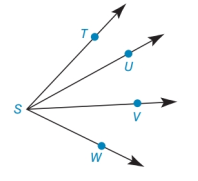Chapter 1.6, Problem 25E### Elementary Geometry for College St...

6th Edition
Daniel C. Alexander + 1 other
ISBN: 9781285195698

#### Solutions

Chapter
Section### Elementary Geometry for College St...

6th Edition
Daniel C. Alexander + 1 other
ISBN: 9781285195698
Textbook Problem
1 views

# Prove the Extended Angle Addition Property by using the Drawing, the Given, and the Prove that follow. Given: ∠ T S W with S U → and S V → Prove: m ∠ T S W = m ∠ T S U + m ∠ U S V + m ∠ V S WTo determine

To prove:

Explanation

Given:

It is given that TSW with SU and SV.

The given figure is,

Approach:

Angle Addition Property is defined as,

If a point X lies in the interior of an angle ABC, then mABX+mXBC=mABC.

Calculation:

Use Angle Addition Property for given angle TSW.

mTSW=mTSU+mUSW(1)

Use Angle Addition Property for given angle USW

### Still sussing out bartleby?

Check out a sample textbook solution.

See a sample solution

#### The Solution to Your Study Problems

Bartleby provides explanations to thousands of textbook problems written by our experts, many with advanced degrees!

Get Started

#### Calculate y'. 5. y = x2 sin x

Single Variable Calculus: Early Transcendentals, Volume I

#### 6x2(3x2+2)+14(x+2)

Applied Calculus for the Managerial, Life, and Social Sciences: A Brief Approach

#### True or False: is a convergent series.

Study Guide for Stewart's Multivariable Calculus, 8th